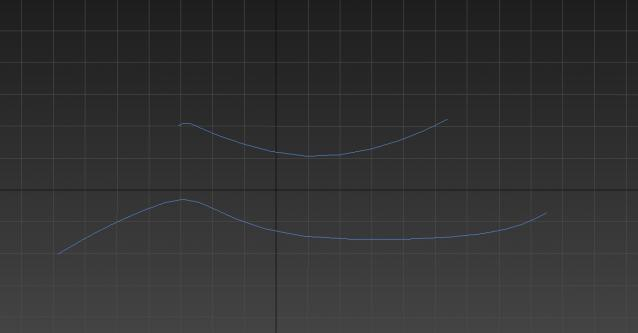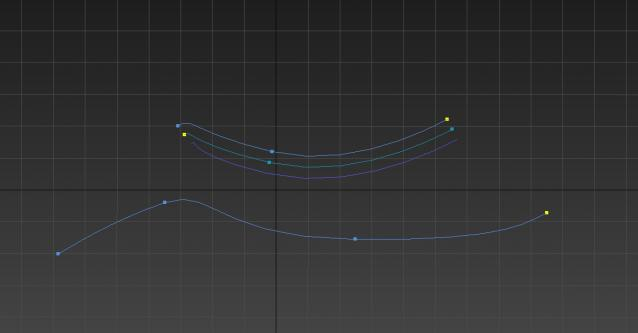# question : parentheses strange behavior

hello i was trying to automate spline outline multiple times. this is the code:

` aao = \$`

` w = 3.5`

` fn deleteoffset spl = (`

` convertToSplineShape spl`

` subobjectLevel = 2`

` todel = numSegments spl 1`

` todel2= todel/2`

` sel = #()`

` setSegSelection spl 1 #()`

` for i = 1 to todel2 do (`

` append sel i `

`)`

` append sel todel`

` setSegSelection spl 1 sel`

` splineOps.delete spl`

` updateshape spl `

`)`

` aa = copy aao`

` spl = getSplineSelection aa`

` allspl = numSplines aa `

`for i = allspl to 1 by -1 do (`

` if i != spl do (`

` deleteSpline aa i`

` )`

` ) `

`applyOffset aa w`

` select aa `

`deleteoffset aa`

` aa2 = copy aa `

`applyOffset aa2 (w *-1)`

` select aa2`

` deleteoffset aa2 `this is the resault of the code above but when i add parentheses to this section of the code this is the result :

` (`

` applyOffset aa w`

` select aa`

` deleteoffset aa`

` aa2 = copy aa`

` applyOffset aa2 (w *-1)`

` select aa2`

` deleteoffset aa2`

` )`which is annoying i cant put that part of the code in for loop or if condition or in macroscript ...

please could some one explain this behavior and suggest a fix .

thanks